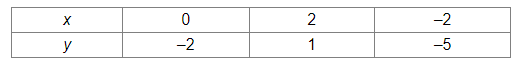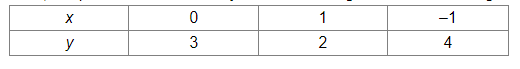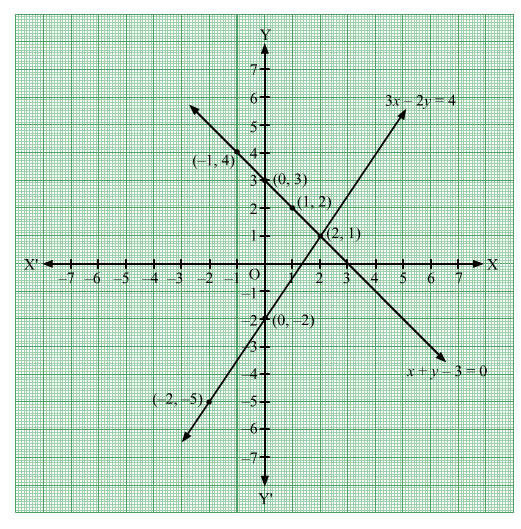# Draw the graphs of the equations 3x – 2y = 4 and x + y – 3 = 0. On the same graph paper,

Question:

Draw the graphs of the equations 3x – 2y = 4 and x + y – 3 = 0. On the same graph paper, find the coordinates of the point were the two graph lines intersect.

Solution:

$3 x-2 y=4$

$\Rightarrow 2 y=3 x-4$

$\Rightarrow y=\frac{3 x-4}{2}$

When $x=0, y=\frac{3 \times 0-4}{2}=\frac{0-4}{2}=\frac{-4}{2}=-2$

When $x=2, y=\frac{3 \times 2-4}{2}=\frac{6-4}{2}=\frac{2}{2}=1$

When $x=-2, y=\frac{3 \times(-2)-4}{2}=\frac{-6-4}{2}=\frac{-10}{2}=-5$

Thus, the points on the line 3x – 2y = 4 are as given in the following table:Plotting the points (0, –2), (2, 1) and (–2–5) and drawing a line passing through these points, we obtain the graph of of the line 3x – 2y = 4.

$x+y-3=0$

$\Rightarrow y=-x+3$

When $x=0, y=-0+3=3$

When $x=1, y=-1+3=2$

When $x=-1, y=-(-1)+3=1+3=4$

Thus, the points on the line x + y – 3 = 0 are as given in the following table:Plotting the points (0, 3), (1, 2) and (–14) and drawing a line passing through these points, we obtain the graph of of the line x + y – 3 = 0.It can be seen that the lines 3x – 2y = 4 and x + y – 3 = 0 intersect at the point (2, 1).